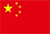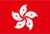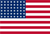Scanning QR Code• Home
• News
• Courses
• Help Center
• Language
•••EN

Why the latest price of Hong Kong stocks is not equal to the latest transaction price

The latest price of Hong Kong stocks is the data provided directly by the exchange. There are different rules of calculation at different stages.

1. Opening bidding stage

1.1 if there is a reference equilibrium price, the current price = the reference equilibrium price (specified by the exchange)

1.2 if there is no reference to the equilibrium price, it will be equivalent to the closing price yesterday.

2.1 if the stock is traded on a case-by-case basis, it is calculated as follows:

● if you buy a price > the last transaction price, the current price = buy a price

● if the first price is less than the last transaction price, the current price = the first price

● other cases, current price = last transaction price

2.2 if the securities are not traded on a case-by-case basis, they are calculated as follows:

● if you buy a price > close yesterday, the current price = buy a price

● if the first price is sold < the price closed yesterday, the current price = the first price

● other cases of pre-price = closing price yesterday

Back to the Top